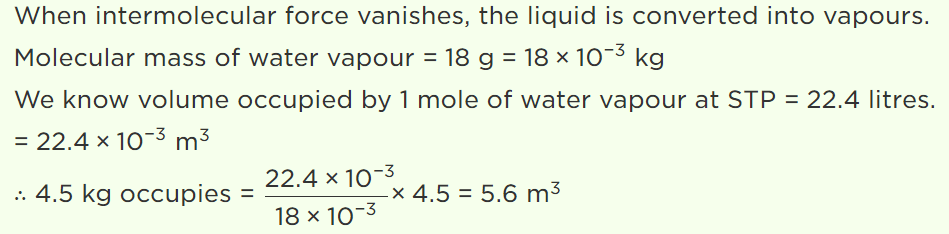## Kinetic Theory Questions and Answers Part-29

1. A closed vessel contains 8gm of oxygen and 7gm of nitrogen. The total pressure is 10 atm at a given temperature. If now oxygen is absorbed by introducing a suitable absorbent the pressure of the remaining gas in atm will be
a) 2
b) 10
c) 4
d) 5

Explanation:2. $CO_{2}\left(O-C-O\right)$     is a triatomic gas. Mean kinetic energy of one gram gas will be (If N-Avogadro's number, k-Boltzmann's constant and molecular weight of $CO_{2}=44$   )
a) (3 / 88)NkT
b) (5 / 88)NkT
c) (6 / 88)NkT
d) (7 / 88)NkT

Explanation:3. 40 calories of heat is needed to raise the temperature of 1 mole of an ideal monoatomic gas from 20°C to 30°C at a constant pressure. The amount of heat required to raise its temperature over the same interval at a constant volume ( R = 2 calorie $mole^{-1}K^{-1}$   ) is
a) 20 calorie
b) 40 calorie
c) 60 calorie
d) 80 calorie

Explanation:4. The pressure and volume of saturated water vapour are P and V respectively. It is compressed isothermally thereby volume becomes V/2, the final pressure will be
a) More than 2P
b) P
c) 2P
d) 4P

Explanation: The pressure and volume of saturated water vapour are P and V respectively. It is compressed isothermally thereby volume becomes V/2, the final pressure will be P

5. If the intermolecular forces vanish away, the volume occupied by the molecules contained in 4.5 kg water at standard temperature and pressure will be
a) $5.6 m^{3}$
b) $4.5 m^{3}$
c) 11.2 litre
d) $11.2 m^{3}$

Explanation:6. When an air bubble of radius ‘r’ rises from the bottom to the surface of a lake, its radius becomes 5r/4 (the pressure of the atmosphere is equal to the 10 m height of water column). If the temperature is constant and the surface tension is neglected, the depth of the lake is
a) 3.53 m
b) 6.53 m
c) 9.53 m
d) 12.53 m

Explanation: The depth of the lake is 9.53 m

7. A horizontal uniform glass tube of 100 cm, length sealed at both ends contain 10 cm mercury column in the middle. The temperature and pressure of air on either side of mercury column are respectively 81°C and 76 cm of mercury. If the air column at one end is kept at 0°C and the other end at 273°C, the pressure of air which is at 0°C is (in cm of Hg)
a) 76
b) 68.2
c) 102.4
d) 122

Explanation: 102.4

8. At standard temperature and pressure the density of a gas is $1.3 kg / m^{3}$  and the speed of the sound in gas is 330 m/sec. Then the degree of freedom of the gas will be
a) 3
b) 4
c) 5
d) 6

Explanation:9. The temperature of 5 moles of a gas which was held at constant volume was changed from 100oC to $120^{\circ} C$  . The change in internal energy was found to be 80 Joules. The total heat capacity of the gas at constant volume will be equal to
a) 8 J/K
b) 0.8 J/K
c) 4.0 J/K
d) 0.4 J/K